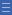## Featured resource

Home > Topdrawer > Fractions > Misunderstandings > Different wholes

# Different wholes

Different wholes

Often students do not realise that when comparing the relative size of fractions, it must be assumed that all fractions refer to the same size whole.

There are two main fraction scenarios: specific and general.

• A fraction that refers to the partitioning of a specific whole describes a particular quantity.

For example, cutting a mini-pizza in half produces a different amount of food than cutting a family-size pizza in half. Although we know $$\frac{1}{2}$$ = $$\frac{1}{2}$$ in a proportional sense, the actual quantities represented by a half are not equal when the 'wholes' in the particular context are not equal.

• A fraction where the actual size of the whole is not relevant is used in a general and abstract sense.

No specific context is required and it is assumed that the 'whole' for all the fractions is of the same size.
For example, logic is sufficient to determine that one-half is larger than one-quarter without needing to know 'half of what?' and 'quarter of what?'

Confusion and misconceptions arise when students do not distinguish between the specific and the general scenarios for using fractions.

Yes

Yes

Name Class SectionShifting wholes Folder 17Fixing wholes Folder 17Cut and find Folder 17